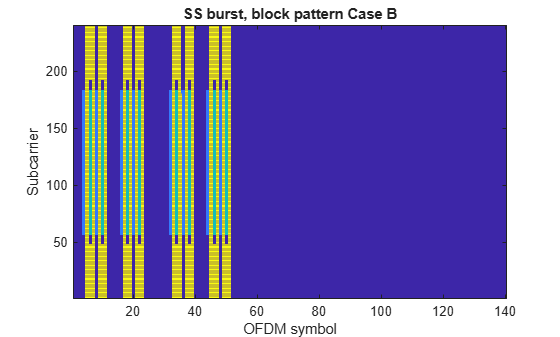# Synchronization Signal Blocks and Bursts

This example shows how to generate a synchronization signal block (SSB) and generate multiple SSBs to form a synchronization signal burst (SS burst). The channels and signals that form a synchronization signal block (primary and secondary synchronization signals, physical broadcast channel) are created and mapped into a matrix representing the block. Finally a matrix representing a synchronization signal burst is created, and each synchronization signal block in the burst is created and mapped into the matrix.

### SS/PBCH block

TS 38.211 Section 7.4.3.1 defines the Synchronization Signal / Physical Broadcast Channel (SS/PBCH) block as 240 subcarriers and 4 OFDM symbols containing the following channels and signals:

• Primary synchronization signal (PSS)

• Secondary synchronization signal (SSS)

• PBCH demodulation reference signal (PBCH DM-RS)

In other documents, for example TS 38.331, the SS/PBCH is termed "synchronization signal block" or "SS block".

Create a 240-by-4 matrix representing the SS/PBCH block:

`ssblock = zeros([240 4])`
```ssblock = 240×4 0 0 0 0 0 0 0 0 0 0 0 0 0 0 0 0 0 0 0 0 0 0 0 0 0 0 0 0 0 0 0 0 0 0 0 0 0 0 0 0 ⋮ ```

#### Primary Synchronization Signal (PSS)

Create the PSS for a given cell identity:

```ncellid = 17; pssSymbols = nrPSS(ncellid)```
```pssSymbols = 127×1 -1 -1 -1 -1 -1 -1 1 1 1 -1 ⋮ ```

`The` variable `pssSymbols` is a column vector containing the 127 BPSK symbols of the PSS.

Create the PSS indices:

`pssIndices = nrPSSIndices;`

The variable `pssIndices` is a column vector of the same size as `pssSymbols`. The value in each element of `pssIndices` is the linear index of the location in the SS/PBCH block to which the corresponding symbols in `pssSymbols` should be mapped. Therefore the mapping of the PSS symbols to the SS/PBCH block can be performed with a simple MATLAB assignment, using linear indexing to select the correct elements of the SS/PBCH block matrix. Note that a scaling factor of 1 is applied to the PSS symbols, to represent ${\beta }_{\mathrm{PSS}}$ in TS 38.211 Section 7.4.3.1.1:

`ssblock(pssIndices) = 1 * pssSymbols;`

Plot the SS/PBCH block matrix to show the location of the PSS:

```imagesc(abs(ssblock)); caxis([0 4]); axis xy; xlabel('OFDM symbol'); ylabel('Subcarrier'); title('SS/PBCH block containing PSS');```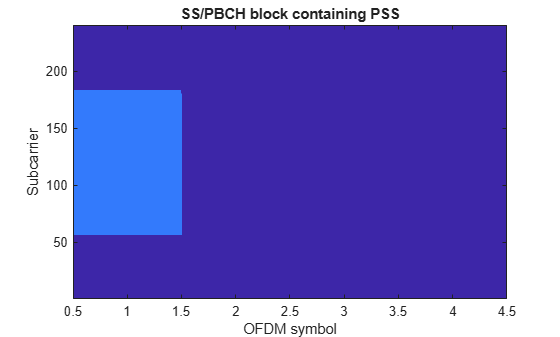#### Secondary Synchronization Signal (SSS)

Create the SSS for the same cell identity as configured for the PSS:

`sssSymbols = nrSSS(ncellid)`
```sssSymbols = 127×1 -1 1 -1 -1 -1 1 -1 1 -1 1 ⋮ ```

Create the SSS indices and map the SSS symbols to the SS/PBCH block, following the same pattern used for the PSS. Note that a scaling factor of 2 is applied to the SSS symbols, to represent ${\beta }_{\mathrm{SSS}}$ in TS 38.211 Section 7.4.3.1.2:

```sssIndices = nrSSSIndices; ssblock(sssIndices) = 2 * sssSymbols;```

The default form of the indices is 1-based linear indices, suitable for linear indexing of MATLAB matrices like `ssblock` as already shown. However, the NR standard documents describe the OFDM resources in terms of OFDM subcarrier and symbol subscripts, using 0-based numbering. For convenient cross-checking with the NR standard, the indices functions accept options to allow the indexing style (linear index versus subscript) and base (0-based versus 1-based) to be selected:

`sssSubscripts = nrSSSIndices('IndexStyle','subscript','IndexBase','0based')`
```sssSubscripts = 127x3 uint32 matrix 56 2 0 57 2 0 58 2 0 59 2 0 60 2 0 61 2 0 62 2 0 63 2 0 64 2 0 65 2 0 ⋮ ```

It can be seen from the subscripts that the SSS is located in OFDM symbol 2 (0-based) of the SS/PBCH block, starting at subcarrier 56 (0-based).

Plot the SS/PBCH block matrix again to show the locations of the PSS and SSS:

```imagesc(abs(ssblock)); caxis([0 4]); axis xy; xlabel('OFDM symbol'); ylabel('Subcarrier'); title('SS/PBCH block containing PSS and SSS');```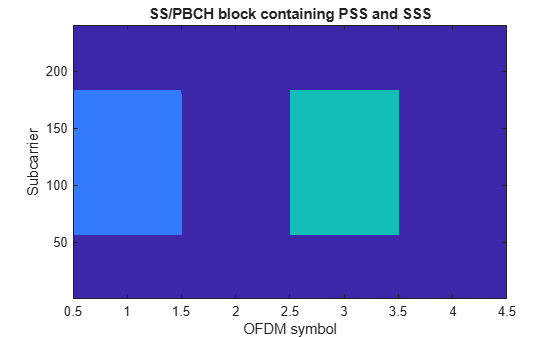The PBCH carries a codeword of length 864 bits, created by performing BCH encoding of the master information block (MIB). For more information on BCH coding, see the functions `nrBCH` and `nrBCHDecode` and their use in the NR Cell Search and MIB and SIB1 Recovery example. Here a PBCH codeword consisting of 864 random bits is used:

`cw = randi([0 1],864,1);`

The PBCH modulation consists of the following steps as described in TS 38.211 Section 7.3.3:

• Scrambling

• Modulation

• Mapping to physical resources

#### Scrambling and modulation

Multiple SS/PBCH blocks are transmitted across half a frame, as described in the cell search procedure in TS 38.213 Section 4.1. Each SS/PBCH block is given an index from $0\dots \mathit{L}-1$, where $\mathit{L}$ is the number SS/PBCH blocks in the half frame. The scrambling sequence for the PBCH is initialized according to the cell identity `ncellid`, and the subsequence used to scramble the PBCH codeword depends on the value $\mathit{v}$, 2 or 3 LSBs of SS/PBCH block index, as described in TS 38.211 Section 7.3.3.1. In this example, $\mathit{v}=0$ is used. The function `nrPBCH` creates the appropriate subsequence of the scrambling sequence, performs scrambling and then performs QPSK modulation:

```v = 0; pbchSymbols = nrPBCH(cw,ncellid,v)```
```pbchSymbols = 432×1 complex -0.7071 + 0.7071i -0.7071 + 0.7071i -0.7071 + 0.7071i -0.7071 - 0.7071i 0.7071 + 0.7071i -0.7071 + 0.7071i -0.7071 + 0.7071i 0.7071 - 0.7071i 0.7071 + 0.7071i 0.7071 + 0.7071i ⋮ ```

#### Mapping to resource elements

Create the PBCH indices and map the PBCH symbols to the SS/PBCH block. Note that a scaling factor of 3 is applied to the PBCH symbols, to represent ${\beta }_{\mathrm{PBCH}}$ in TS 38.211 Section 7.4.3.1.3:

```pbchIndices = nrPBCHIndices(ncellid); ssblock(pbchIndices) = 3 * pbchSymbols;```

Plot the SS/PBCH block matrix again to show the locations of the PSS, SSS and PBCH:

```imagesc(abs(ssblock)); caxis([0 4]); axis xy; xlabel('OFDM symbol'); ylabel('Subcarrier'); title('SS/PBCH block containing PSS, SSS and PBCH');```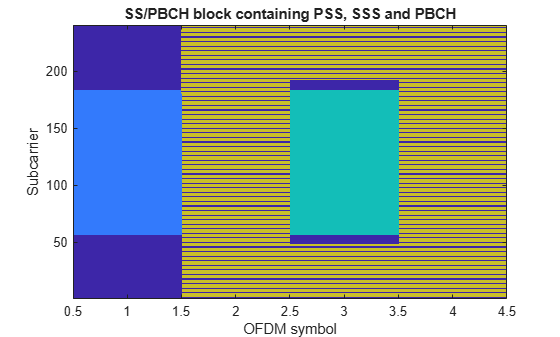#### PBCH Demodulation Reference Signal (PBCH DM-RS)

The final component of the SS/PBCH block is the DM-RS associated with the PBCH. Similar to the PBCH, the DM-RS sequence used derives from the SS/PBCH block index and is configured using the variable ${\stackrel{‾}{\mathit{i}}}_{\mathrm{SSB}}$ described in TS 38.211 Section 7.4.1.4.1. Here ${\stackrel{‾}{\mathit{i}}}_{\mathrm{SSB}}=0$ is used:

```ibar_SSB = 0; dmrsSymbols = nrPBCHDMRS(ncellid,ibar_SSB)```
```dmrsSymbols = 144×1 complex 0.7071 - 0.7071i 0.7071 + 0.7071i -0.7071 + 0.7071i -0.7071 + 0.7071i 0.7071 - 0.7071i 0.7071 + 0.7071i 0.7071 - 0.7071i -0.7071 - 0.7071i -0.7071 - 0.7071i 0.7071 + 0.7071i ⋮ ```

Note that TS 38.211 Section 7.4.1.4.1 defines an intermediate variable ${\mathit{i}}_{\mathrm{SSB}}$ which is defined identically to $\mathit{v}$ described previously for the PBCH.

Create the PBCH DM-RS indices and map the PBCH DM-RS symbols to the SS/PBCH block. Note that a scaling factor of 4 is applied to the PBCH DM-RS symbols, to represent ${\beta \text{\hspace{0.17em}}}_{\mathrm{PBCH}}^{\mathrm{DM}-\mathrm{RS}}$ in TS 38.211 Section 7.4.3.1.3:

```dmrsIndices = nrPBCHDMRSIndices(ncellid); ssblock(dmrsIndices) = 4 * dmrsSymbols;```

Plot the SS/PBCH block matrix again to show the locations of the PSS, SSS, PBCH and PBCH DM-RS:

```imagesc(abs(ssblock)); caxis([0 4]); axis xy; xlabel('OFDM symbol'); ylabel('Subcarrier'); title('SS/PBCH block containing PSS, SSS, PBCH and PBCH DM-RS');```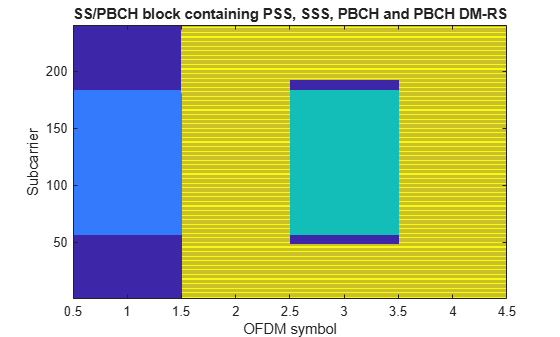### Generating an SS burst

An SS burst, consisting of multiple SS/PBCH blocks, can be generated by creating a larger grid and mapping SS/PBCH blocks into the appropriate locations, with each SS/PBCH block having the correct parameters according to the location.

#### Create SS burst grid

In the NR standard, OFDM symbols are grouped into slots, subframes and frames. As defined in TS 38.211 Section 4.3.1, there are 10 subframes in a frame, and each subframe has a fixed duration of 1ms. Each SS burst has a duration of half a frame, and therefore spans 5 subframes:

`nSubframes = 5`
```nSubframes = 5 ```

TS 38.211 Section 4.3.2 defines each slot as having 14 OFDM symbols (for normal cyclic prefix length) and this is fixed:

`symbolsPerSlot = 14`
```symbolsPerSlot = 14 ```

However, the number of slots per subframe varies and is a function of the subcarrier spacing. As the subcarrier spacing increases, the OFDM symbol duration decreases and therefore more OFDM symbols can be fitted into the fixed subframe duration of 1ms.

There are 5 subcarrier spacing configurations $\mu =0...4$, with the corresponding subcarrier spacing being $15\cdot {2}^{\mu }$ kHz. In this example we shall use $\mu =1$, corresponding to 30 kHz subcarrier spacing:

`mu = 1`
```mu = 1 ```

The number of slots per subframe is ${2}^{\mu }$, as doubling the subcarrier spacing halves the OFDM symbol duration. Note that definition of a slot in NR is different from LTE: a subframe in LTE consists of 2 slots of 7 symbols (for normal cyclic prefix) whereas in NR, a subframe using the LTE subcarrier spacing ($\mu =0$, 15 kHz) consists of 1 slot of 14 symbols.

Calculate the total number of OFDM symbols in an SS burst:

`nSymbols = symbolsPerSlot * 2^mu * nSubframes`
```nSymbols = 140 ```

Create an empty grid for the whole SS burst :

`ssburst = zeros([240 nSymbols]);`

#### Define SS block pattern

The pattern of SS/PBCH blocks within an SS burst is indirectly specified by the cell search procedure in TS 38.213, which describes the locations in which the UE may detect an SS/PBCH block. There are 5 block patterns, Case A - Case E, which have different subcarrier spacings and are applicable for different carrier frequencies.

Create the indices of the first symbols in the candidate SS/PBCH blocks for block pattern Case B, which has $\mathit{L}=8$ blocks per burst:

```n = [0, 1]; firstSymbolIndex = [4; 8; 16; 20] + 28*n; firstSymbolIndex = firstSymbolIndex(:).'```
```firstSymbolIndex = 1×8 4 8 16 20 32 36 44 48 ```

#### Create SS burst content

Now a loop can be created which generates each SS block and assigns it into the appropriate location of the SS burst. Note the following:

• The code re-uses various variables created earlier in this example (PSS, SSS, and 4 sets of indices)

• The PSS and SSS are independent of the SS/PBCH block index, so can be mapped into the SS block before the loop

• The PBCH indices and PBCH DM-RS indices are independent of the SS/PBCH block index, so do not need updated in the loop

• ${\mathit{i}}_{\mathrm{SSB}}$, ${\stackrel{‾}{\mathit{i}}}_{\mathrm{SSB}}$ and $\mathit{v}$ are set up according to the rules in TS 38.211 Sections 7.3.3.1 and 7.4.1.4.1 for the case of $\mathit{L}=8$.

• Each channel / signal has been scaled in order to give them different colors in the final plot

```ssblock = zeros([240 4]); ssblock(pssIndices) = pssSymbols; ssblock(sssIndices) = 2 * sssSymbols; for ssbIndex = 1:length(firstSymbolIndex) i_SSB = mod(ssbIndex - 1,8); ibar_SSB = i_SSB; v = i_SSB; pbchSymbols = nrPBCH(cw,ncellid,v); ssblock(pbchIndices) = 3 * pbchSymbols; dmrsSymbols = nrPBCHDMRS(ncellid,ibar_SSB); ssblock(dmrsIndices) = 4 * dmrsSymbols; ssburst(:,firstSymbolIndex(ssbIndex) + (0:3)) = ssblock; end```

Finally, plot the SS burst content:

```imagesc(abs(ssburst)); caxis([0 4]); axis xy; xlabel('OFDM symbol'); ylabel('Subcarrier'); title('SS burst, block pattern Case B');```